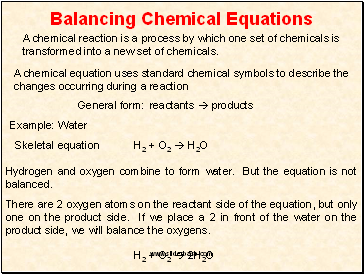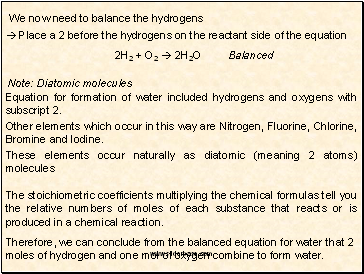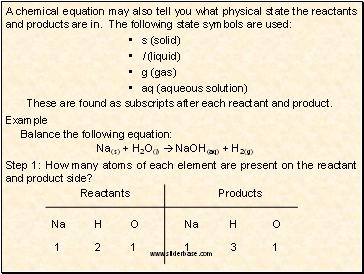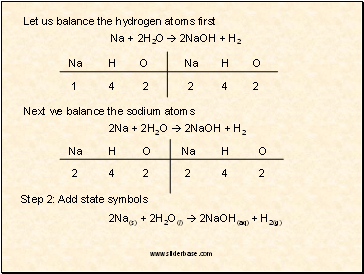# Balancing Chemical Equations. Balancing redox equationsPage 1

#### WATCH ALL SLIDES

Slide 1## Balancing Chemical Equations

A chemical reaction is a process by which one set of chemicals is

transformed into a new set of chemicals.

A chemical equation uses standard chemical symbols to describe the changes occurring during a reaction

General form: reactants  products

Example: Water

Skeletal equation

H2 + O2  H2O

Hydrogen and oxygen combine to form water. But the equation is not balanced.

There are 2 oxygen atoms on the reactant side of the equation, but only one on the product side. If we place a 2 in front of the water on the product side, we will balance the oxygens.

H2 + O2  2H2O

Slide 2We now need to balance the hydrogens

 Place a 2 before the hydrogens on the reactant side of the equation

2H2 + O2  2H2O

Balanced

Note: Diatomic molecules

Equation for formation of water included hydrogens and oxygens with subscript 2.

Other elements which occur in this way are Nitrogen, Fluorine, Chlorine, Bromine and Iodine.

These elements occur naturally as diatomic (meaning 2 atoms) molecules

The stoichiometric coefficients multiplying the chemical formulas tell you the relative numbers of moles of each substance that reacts or is produced in a chemical reaction.

Therefore, we can conclude from the balanced equation for water that 2 moles of hydrogen and one mol of oxygen combine to form water.

Slide 3A chemical equation may also tell you what physical state the reactants and products are in. The following state symbols are used:

s (solid)

l (liquid)

g (gas)

aq (aqueous solution)

These are found as subscripts after each reactant and product.

Example

Balance the following equation:

Na(s) + H2O(l)  NaOH(aq) + H2(g)

Step 1: How many atoms of each element are present on the reactant and product side?

Reactants

Products

Na

H

O

1

2

1

Na

H

O

1

3

1

Slide 4Let us balance the hydrogen atoms first

Na + 2H2O  2NaOH + H2

Na

H

O

Na

H

O

1

4

2

2

4

2

Next we balance the sodium atoms

2Na + 2H2O  2NaOH + H2

Na

H

O

Na

H

O

2

4

2

2

4

2

2Na(s) + 2H2O(l)  2NaOH(aq) + H2(g)

Slide 5Questions

Balance the following equations:

(a) P2O5(s) + H2O(l)  H3PO4(aq)

Answer: P2O5(s) + 3H2O(l)  2H3PO4(aq)

Go to page:
1  2  3  4  5  6  7  8